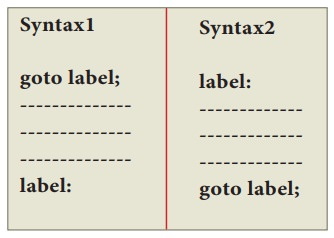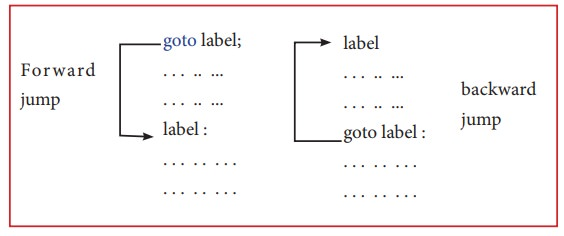Home | | Computer Science 11th std | C++ goto statement

# C++ goto statement

The goto statement is a control statement which is used to transfer the control from one place to another place without any condition in a program.

goto statement

The goto statement is a control statement which is used to transfer the control from one place to another place without any condition in a program.

The syntax of the goto statement is;In the syntax above, label is an identifier. When goto label; is encountered, the control of program jumps to label: and executes the code below it.

## Illustration 10.18 C++ program to calculate average of given numbers using goto statement

#include <iostream>

using namespace std;

int main()

{

float num, average, sum = 0.0;

int i, n;

cout<< "Maximum number of inputs: ";

cin>> n;

for(i = 1; i<= n; ++i)

{

cout<< "Enter n" <<i<< ": ";

cin>>num;

if(num< 0.0)

{

// Control of the program move to jump: goto jump;

}

sum += num;

}

jump:

average = sum / (i - 1);

cout<< "\nAverage = " << average; return 0;

}

### Output

Maximum number of inputs: 5

Enter n1: 10

Enter n2: 20

Enter n3: -2 Average = 15

In the above program the average of numbers entered by the user is calculated. If the user enters a negative number, it is ignored the average of numbers entered. Until that point is calculated.

Study Material, Lecturing Notes, Assignment, Reference, Wiki description explanation, brief detail
11th Computer Science : Chapter 10 : Flow of Control : C++ goto statement |